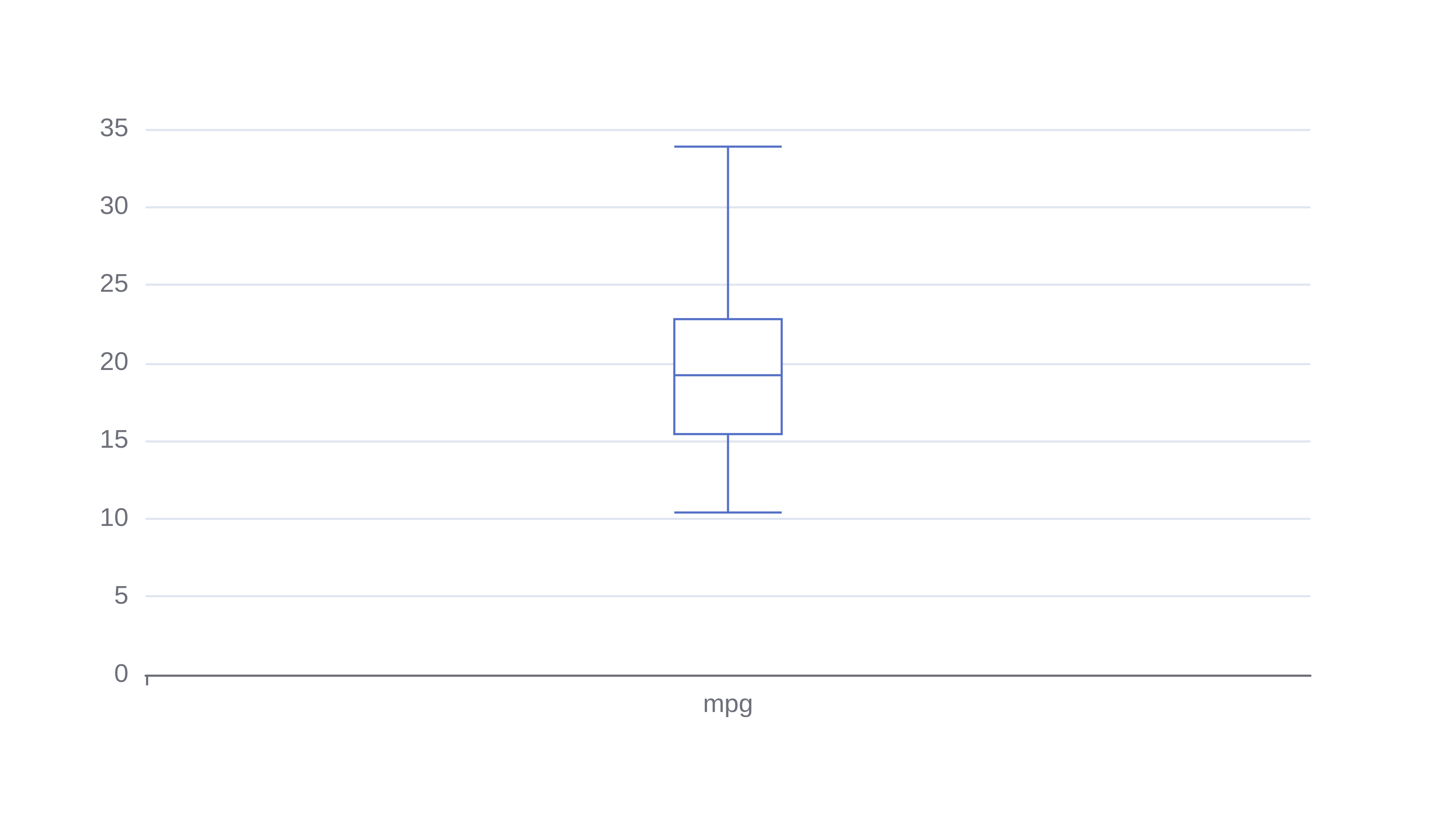# Box and Whisker

Or input your data as csv

Sharing helps us build more free tools

Create a box and whisker plot with this free online box and whisker plot maker.

Box plots are an easy way to view distributions of numerical data using the five-number summary.

The five-number summary tells us the critical points of a whole dataset: the minimum, first quartile, median, third quartile, and maximum values.

The box and whisker plot below shows the miles per gallon fuel efficiency of different cars in the mt cars data set.## When To Use aBox plot

Use a box and whisker plot when you want to summarize a distribution. For example, you could use a boxplot to view the maximum temperatures over the last 3 months.

If you want to take a closer look at the distribution, then use a histogram. Histograms perform less aggregation to raw data than boxplots. So they are a useful tool for seeing a distribution's shape.

Boxplots are also useful for comparing distributions. For example, if you wanted to compare the max temperatures of June 2022 to the max temperatures of June 1990 you could use two boxplots side-by-side. One for June 2022 temperatures and one for June 1990 temperatures.

## How to Make a Box and Whisker Plot

Click the dataset input at the top of the page. Or drag and drop your dataset into the input box.

2. ### Pick a column for the x-axis

Choose a continuous variable for best results

## How to Read a Box-and-Whisker Plot

A box and whisker plot has 5 important points.

The bottom line shows the minimum value. Sometimes it might show a 5th percentile so that low outliers are removed.

The bottom side of the central box shows the first quartile of the data distribution. 25% of all values are below the 25th percentile.

The line in the middle of the box part shows the median of the data set. The median measures the "middle value". It is less sensitive to noise than the mean value.

The top side of the central box shows the third quartile. 75% of all values are below the third quartile.

The difference between the first and third quartile is called the interquartile range. The interquartile range measures where the middle 50% of values lie.

The very top line shows the maximum value. Sometimes it might instead show a 5th percentile so that high outliers are removed.

## How do I find the interquartile range of a box plot?

The interquartile range can be found by subtracting the first quartile from the third quartile. IQR = Q3 - Q1.

The interquartile range is always positive because the third quartile is always greater than or equal to the first quartile.